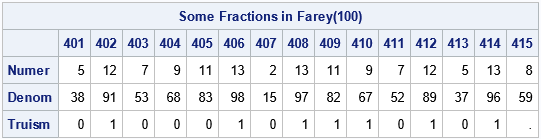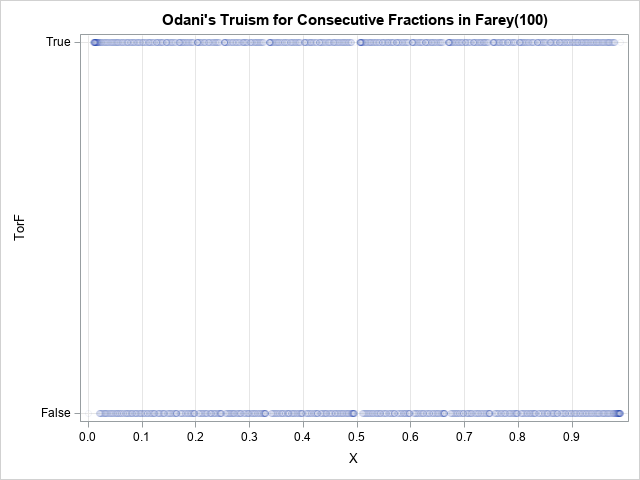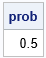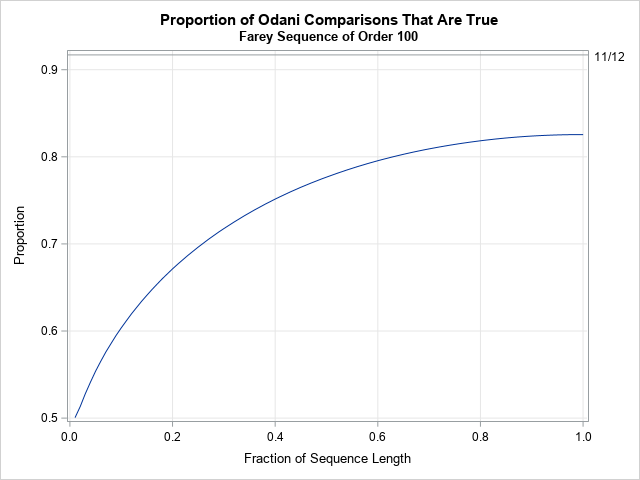Odani's truism is a mathematical result that says that if you want to compare the fractions a/b and c/d, it often is sufficient to compare the sums (a+d) and (b+c) rather than the products a*d and b*c. (All of the integers a, b, c, and d are positive.) If you compare the products, you will know the correct answer 100% of the time because a/b < c/d if and only if a*d < b*c. However, K. Odani proved (1998) that the expression (a+d) < (b+c) is true 91% of the time when the integers a, b, c, and d are chosen uniformly at random in the interval [1,N], where N is a very large integer. A previous article shows a Monte Carlo simulation that demonstrates Odani's truism. The article also discusses John D. Cook's suggestion that "it would be interesting to try to assess how often the rule of thumb presented here is correct in practice."

As discussed at the end of the previous article, the 91% probability includes many fractions that are easy to compare in your head, such as 6/29 versus 35/18, and 1/6 versus 5/8. Odani's result also includes unreduced fractions such as 2/4 and 3/6. What is the probability that comparing the sums gives the correct answer if you consider only reduced fractions a/b and c/d in the interval [0,1] where the fractions are close to each other? That is the subject of this article. Briefly, this article examines whether Odani's truism has any value in practice.

Given a pair of fractions a/b < c/d, we say that Odani's truism is "true" (or valid or correct) if (a+d) < (b+c). Otherwise, we say that the truism is "false."

### The Farey sequence

I will use the Farey sequence in this investigation. The Farey sequence of order n is the sequence of all reduced fractions on [0,1] whose denominators do not exceed n, listed in increasing order. In practice, we do not usually need to compare fractions with huge denominators. Most of the time, we encounter fractions whose denominators are less than 100, which corresponds to the Farey sequence of order 100.

Consider the Farey sequence of order n for a small value of n, such as n=100. The sequence is in increasing order, so you can compare each term of the sequence with the next k terms and compute how often Odani's truism is correct. When k is small compared to n, this method estimates how often Odani's truism is valid for fractions in [0,1] that are near each other.

### First attempt: Fractions that are adjacent in the Farey seqence

Before looking a the general case of k > 0, let's look at the special case where k=1. For each fraction in the Farey sequence, a/d, let c/d be the next fraction in the Farey sequence. Because the Farey sequence is in order, we know that a/b < c/d. Compute the Odani sum (a+d)-(b+c). If the sum is negative, then Odani's truism is correct for that a/b and c/d. Otherwise, the truism is false.

The following SAS/IML function computes the Farey sequence for any order n:

```proc iml; /* Generate Farey sequence of order n: all reduced fractions on [0,1] whose denominators do not exceed n, listed in increasing order https://blogs.sas.com/content/iml/2021/03/17/farey-sequence.html */ start Farey(n); /* upperbound on sequence length is n*(n+3)/2. See https://oeis.org/A005728 */ F = j(2, max(2,n*(n+3)/2), .); F[,1] = {0, 1}; F[,2] = 1 // n; order = 2; a=0; b=1; c=1; d=n; /* fractions are a/b and c/d */ do while (d > 1); order = order+1; k = floor((n + b)/d); /* integer division */ a_prev = a; a = c; b_prev = b; b = d; c = k*c - a_prev; d = k*d - b_prev; F[,order] = c // d; end; /* remove any unassigned elements */ keepidx = 1:order; return( F[ ,keepidx] ); finish;```

You can call the function generate the Farey sequence for n=100. Let's print out a few fractions in the sequence along with an indicator variable that shows whether their Odani sums are negative:

```S = Farey(100); /* generaste Farey sequence of order n=100 */   idx = 401:415; /* look at a few terms in the Farey sequence */ s0 = S[,idx]; /* get the corresponding fractions */ /* evaluate the Odani sum on adjacent pairs */ i = 1:ncol(idx)-1; a = S0[1,i]; b = S0[2,i]; /* first row of S contains numerators; second row contains denominators */ c = S0[1,i+1]; d = S0[2,i+1]; OdaniSum = (a+d)-(b+c); /* when sum is negative, the truism is true (predicts that a/b < c/d) */ Truism = (OdaniSum < 0); /* In SAS/IML 15.1, you can use numeric value for the COLNAME= option. See https://blogs.sas.com/content/iml/2019/07/31/numeric-column-header-print.html */ print (s0//(Truism||.))[L="Some Fractions in Farey(100)" c=idx r={"Numer" "Denom" "Truism"}];```The Farey sequence of order 100 has 3,045 elements. The table shows the values for the elements near the fraction 2/15, which is the 407th element in the sequences. The program compares the i_th fraction to the (i+1)st fraction and computes whether (a+d) < (b+c) for the adjacent pairs. For the 14 comparisons in this example, the Odani truism was true for seven and false for the other seven.

A 50% success rate is not very good! Maybe fractions near 2/15 are "unusual" in some way? Let's compare all adjacent pairs of fractions in the Farey(100) sequence and compute the proportion that satisfies Odani's truism. We can also plot the truth or falsity of Odani's truism for each fraction in the Farey sequence:

```/* compare each fraction to the one that follows it */ i = 1:ncol(S)-1; /* the indices of a/b; i+1 contains the indices of c/d */ a = S[1,i]; b = S[2,i]; c = S[1,i+1]; d = S[2,i+1]; Truism = ((a+d)-(b+c) < 0);   /* plot the result (T or F) versus the fraction */ title "Odani's Truism for Consecutive Fractions in Farey(100)"; x = S[1,i] / S[2,i]; TorF = choose(Truism=1, "True ", "False"); call scatter(x, TorF) grid={x y} option="transparency=0.95" xvalues=do(0,1,0.1);   prob = mean(Truism`); print prob;```When applied to adjacent fractions in the Farey sequence, Odani's truism is true only 50% of the time. The scatter plot shows the truth or falsehood of Odani's truism for each fraction in the Farey sequence. The results do not seem to depend on the location of the fractions within the interval [0,1].

Notice that this result does not violate Odani's theorem because we are comparing only certain fractions: reduced fractions in [0,1] that are adjacent to each other in the Farey sequence.

### Compare fractions that are k terms away in the Farey seqence

For a Farey sequence that contains L terms, the previous section shows how to compare L-1 pairs of fractions. Each fraction was compared to the next fraction in the Farey sequence. If we perform ALL pairwise comparisons of the terms in the Farey sequence, Odani's truism will be valid for a larger proportion of comparisons. It probably won't hold for 91% of the comparisons (because we are dealing with reduced fractions in [0,1]), but I expect it to hold for more than 50%.

Let L be the length of the Farey sequence. The following function compares each term in the Farey sequence with the next k terms in the sequence, when possible. For the first L-k terms, you can compare each term with the k terms that follow. For the last k terms, you can only compare to the end of the sequence. Thus, there are a total of k*(L-k) + k*(k-1)/2 comparisons. If you count the number of pairs that satisfy Odani's truism and divide by the total number of comparisons, you get the proportion of comparisons for which Odani's sum accurately predicts whether one fraction is smaller than another:

```/* S is a Farey sequence. k is the number of subsequent terms that we compare to each fraction. For example: k = 1 will compare adjacent terms k = 2 will compare each fraction with the following two terms, and so on... */ start CompareFract(S, k); Len = ncol(S); count = 0; numCompared = k*(Len-k) + k*(k-1)/2; /* total number of pairwise comparisons */ do i = 1 to Len-1; a = S[1,i]; b = S[2,i]; /* i = the indices of a/b; /* we know that a/b is less than all the fractions that follow */ idx = (i+1):min(i+k, Len); /* the next 'k' fractions or until the end */ c = S[1, idx]; d = S[2, idx]; /* c/d for the next k fractions */ OdaniSum = (a+d) - (b+c); count = count + sum(OdaniSum<0); /* how many fractions satisfy the eqn? */ end; prop = count / numCompared; /* proportion of comparisons that satisfy eqn */ return count || numCompared || prop; finish;```

Let's apply the function to the Farey sequence of order 100, which has 3,045 terms. Let's perform a total of 100 comparisons, where k is evenly distributed among the values 1–3045. (The number of comparisons, 100, is unrelated to the order of the Farey sequence.) The following statements call the CompareFracts function for values of k that are approximately 3045/100 units apart. The exact values are k={30, 60, 91, ..., 3014, 3045}.

```S = Farey(100); pct = do(0.01, 1.0, 0.01); /* evenly spaced percentages */ k = int( ncol(S)*pct ); /* choose k to be percentage of sequence length */ results = j(ncol(k), 3, .); do i = 1 to ncol(k); results[i,] = CompareFract(S, k[i]); end;   title "Proportion of Odani Comparisons That Are True"; title2 "Farey Sequence of Order 100"; refStmt = "refline 0.917 / axis=y noclip label='11/12';"; call series(pct, results[,3]) grid={x y} other=refStmt label={'Fraction of Sequence Length' 'Proportion'};```The graph shows the percentage of comparisons for which Odani's truism is valid when comparing nearby fractions in the Farey sequence of order 100. The horizontal axis displays the proportion of the sequence that is being compared: 1%, 2%, ..., 100%. The left side of the graph indicates that if you compare each fraction to a small number of fractions that are close to it (such as 1% of the sequence length), Odani's truism is valid only about 50% of the time. If you compare each fraction to more fractions (which are, by definition, farther away from it), then Odani's truism is correct for a larger proportion of comparisons. If you consider all pairwise comparisons of the fractions, you find that about 82.5% of the comparisons satisfy Odani's truism (for the fractions in this Farey sequence).

A similar curve is seen when you consider larger sets of fractions. For example, for the Farey sequence of order 500 (which contains the 76,117 fractions whose denominators are less than or equal to 500), the graph looks similar except it is slightly higher: when you compare all fractions, Odani's truism is valid 83.2% of the time. As you consider larger Farey sequences, the proportion of comparisons for which Odani's truism increases very slowly. For the Farey sequence of order 1000, which has 304,193 terms, Odani's truism is valid 83.3% of the time when you perform all pairwise comparisons. Warning: Performing all pairwise comparisons for the Farey(1000) sequence is expensive! It takes about 30 minutes when using the program in this article to compare the 46 billion pairs of fractions. It is interesting that 83.3% ≈ 5/6.

### Summary

Odani's truism is a surprising mathematical theorem that says you can often determine whether a/b < c/d by comparing the sums (a+d) and (b+c). Although Odani's truism is correct 91% of the time (asymptotically) when you consider all integers a, b, c, and d that are less than some large number N, the truism is not very useful in practice. In practice, you often want to compare reduced fractions that are close to each other. By using Farey sequences, this article demonstrates that Odani's truism is correct only about 50% of the time when you compare a fraction and its nearby neighbors in the Farey sequence. It is only when you consider ALL pairwise comparisons of fractions that Odani's truism is correct for a large percentage of the comparisons. When comparing all reduced fractions whose denominators are 1000 or less, Odani's truism is correct about 83.3% of the time.

Share